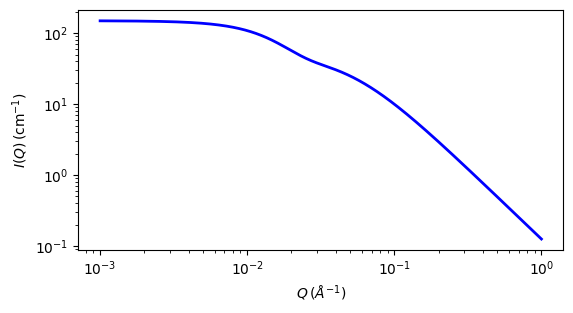# gauss_lorentz_gel

Gauss Lorentz Gel model of scattering from a gel structure

Parameter

Description

Units

Default value

scale

Scale factor or Volume fraction

None

1

background

Source background

cm-1

0.001

gauss_scale

Gauss scale factor

None

100

cor_length_static

Static correlation length

100

lorentz_scale

Lorentzian scale factor

None

50

cor_length_dynamic

Dynamic correlation length

20

The returned value is scaled to units of cm-1 sr-1, absolute scale.

This model calculates the scattering from a gel structure, but typically a physical rather than chemical network. It is modeled as a sum of a low-q exponential decay (which happens to give a functional form similar to Guinier scattering, so interpret with care) plus a Lorentzian at higher-q values. See also the gel_fit model.

Definition

The scattering intensity $$I(q)$$ is calculated as (Eqn. 5 from the reference)

$I(q) = I_G(0) \exp(-q^2\Xi ^2/2) + I_L(0)/(1+q^2\xi^2)$

$$\Xi$$ is the length scale of the static correlations in the gel, which can be attributed to the “frozen-in” crosslinks. $$\xi$$ is the dynamic correlation length, which can be attributed to the fluctuating polymer chains between crosslinks. $$I_G(0)$$ and $$I_L(0)$$ are the scaling factors for each of these structures. Think carefully about how these map to your particular system!

Note

The peaked structure at higher $$q$$ values (Figure 2 from the reference) is not reproduced by the model. Peaks can be introduced into the model by summing this model with the gaussian_peak model.

For 2D data the scattering intensity is calculated in the same way as 1D, where the $$q$$ vector is defined as

$q = \sqrt{q_x^2 + q_y^2}$Fig. 103 1D plot corresponding to the default parameters of the model.

Source

gauss_lorentz_gel.py

References

1. G Evmenenko, E Theunissen, K Mortensen, H Reynaers, Polymer, 42 (2001) 2907-2913

Authorship and Verification

• Author: